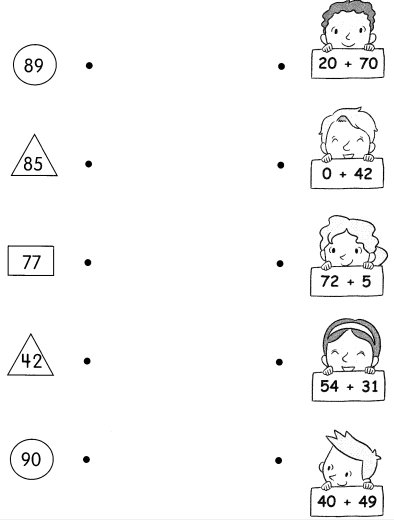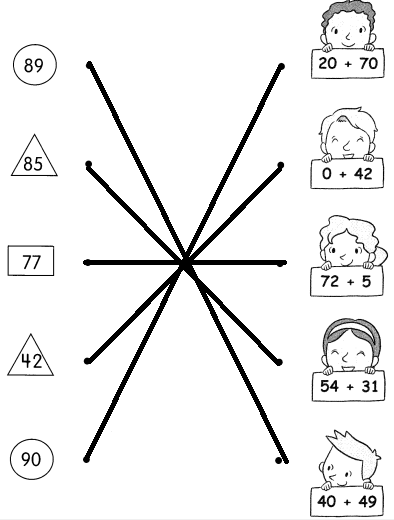Example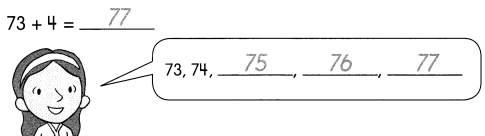Question 1.
85 + 3 = ____________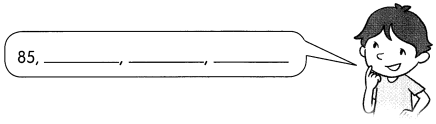85 + 3 = 88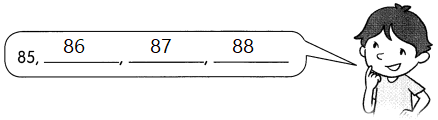Explanation:
85 + 3
When add 1 to the a number the sum is number after.
Add by counting on increasing 1  to the given addend as shown above,
till we get the sum.
85,86,87, and 88.

Question 2.
62 + 6 = _____________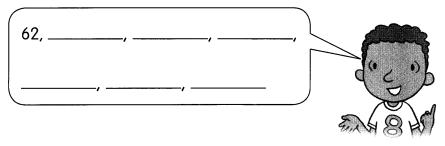62 + 6 = 68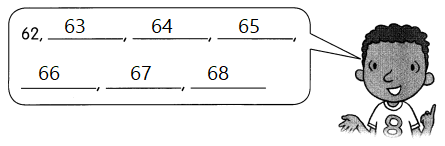Explanation:
62 + 6
When add 1 to the a number the sum is number after.
Add by counting on increasing 1  to the given addend as shown above,
till we get the sum.
62,63,64,65,66,67 and 68.

Question 3.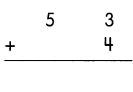Explanation:
Add 4 to 53 to get the sum
So, 53 + 4 = 57

Question 4.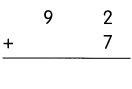Explanation:
Add 7 to 92 to get the sum
So, 92 + 7 = 99

Question 5.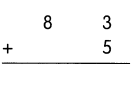Explanation:
Add 5 to 83 to get the sum
So, 80 + 8 = 88

Question 6.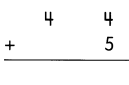Explanation:
Add 9 to 40 to get the sum
So, 40 + 9 = 49

Question 7.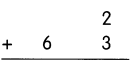Explanation:
Add 5 to 60 to get the sum
So, 60 + 5 = 65

Question 8.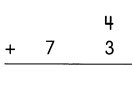Explanation:
Add 7 to 77 to get the sum
So, 70 + 7 = 77

Fill in the missing numbers.

Question 9.
5 + 82 = ____________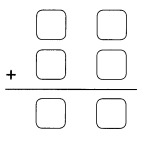5 + 82 = 87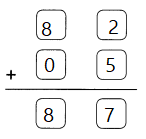Explanation:
Write the addends individually in their place values,
then regroup the ones
2 + 5 = 7
Add the regrouping ones value to tens
80 + 7 = 87

Question 10.
93 + 2 = _____________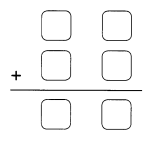93 + 2 = 95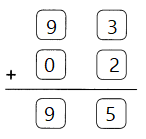Explanation:
Write the addends individually in their place values,
then regroup the ones
2 + 3 = 5
Add the regrouping ones value to tens
90 + 5 = 87

Question 11.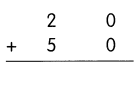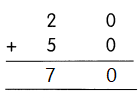Explanation:
The sum is always greater than the numbers being added.
When we change the order of numbers while adding, the sum does not change.
20 + 50 = 70

Question 12.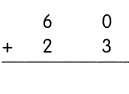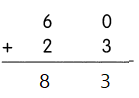Explanation:
The sum is always greater than the numbers being added.
When we change the order of numbers while adding, the sum does not change.
60 + 23 = 83

Question 13.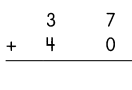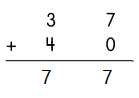Explanation:
The sum is always greater than the numbers being added.
When we change the order of numbers while adding, the sum does not change.
37 + 40 = 77

Question 14.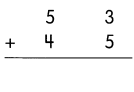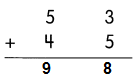Explanation:
The sum is always greater than the numbers being added.
When we change the order of numbers while adding, the sum does not change.
53 + 45 = 98

Question 15.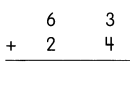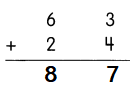Explanation:
The sum is always greater than the numbers being added.
When we change the order of numbers while adding, the sum does not change.
63 + 24 = 87

Question 16.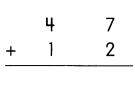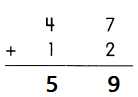Explanation:
The sum is always greater than the numbers being added.
When we change the order of numbers while adding, the sum does not change.
47 + 12 = 59

Fill in the missing numbers.

Question 17.
56 + 23 = ___________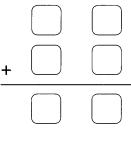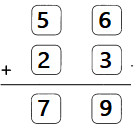Explanation:
The given addends are arranged according to place values.
The sum is always greater than the numbers being added.
When we change the order of numbers while adding, the sum does not change.
56 + 23 = 79

Question 18.
86 + 13 = ___________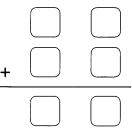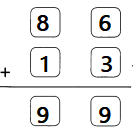Explanation:
The given addends are arranged according to place values.
The sum is always greater than the numbers being added.
When we change the order of numbers while adding, the sum does not change.
86 + 13 = 99

Match.

Question 19.